Topics: AI | Data Science | Data Visualization | Deep Learning | Machine Learning | NLP | Python | R | Statistics

KDnuggets Home » News » 2018 » Oct » Opinions » How I Learned to Stop Worrying and Love Uncertainty ( 18:n41 )

# How I Learned to Stop Worrying and Love Uncertainty

This is a written version of Data Scientist Adolfo Martínez’s talk at Software Guru’s DataDay 2017. There is a link to the original slides (in Spanish) at the top of this post.

### Bayesian Statistics

Bayesianism is rooted in the idea that probability is a measure of uncertainty and, as such, it is dependent on the information available to the individual making the measurement. As a measure, it can be applied to anything you can think of, including unique events, unknown quantities, or the truth about a statement.

The term refers to Thomas Bayes, an 18th-century reverend who proved a special case of the theorem that bears his name. This theorem provides a way to compute the “inverse probability”, that is, the probability of an event Agiven an event B when we know the probability of B given A.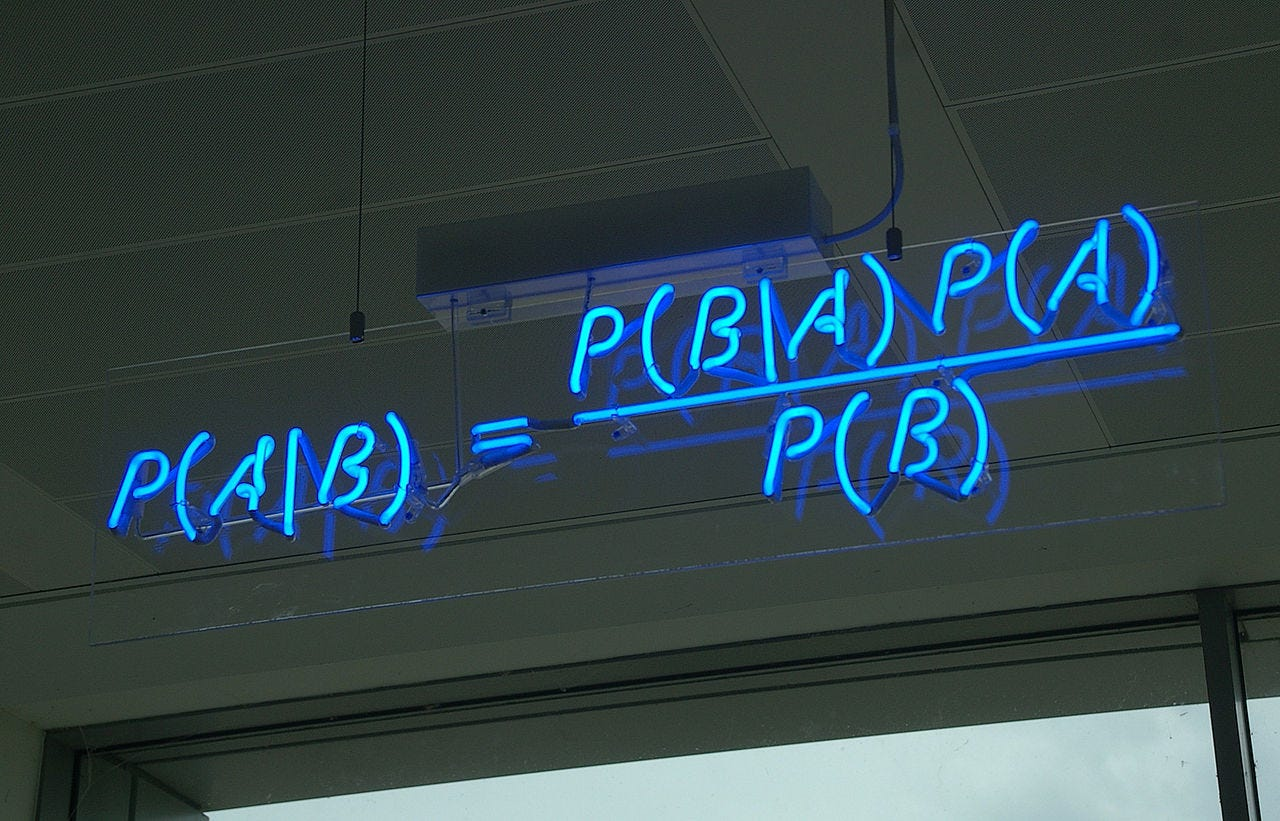Bayes Theorem in a neon sign
For Bayesians, it is a way to make inference about parameters, given the model of the data and the prior distribution of the parameter. This prior distribution encodes the information possessed before any data is observed.

Through the use of this theorem and its definition of probability, Bayesian Statistics can combine information possessed about a phenomenon with observed data about it, and produce updated, more accurate information. And though the inference made in this way is subjective, the theory of Bayesian Statistics states that, as we collect more and more data, the subjective part (the prior information) becomes less and less relevant; the subjective approximates the objective.

Like in frequentism, simple Bayesian models have a straightforward interpretation, for example, the posterior distributions of linear coefficients measure the uncertainty
around the effect of an independent variable in the dependent.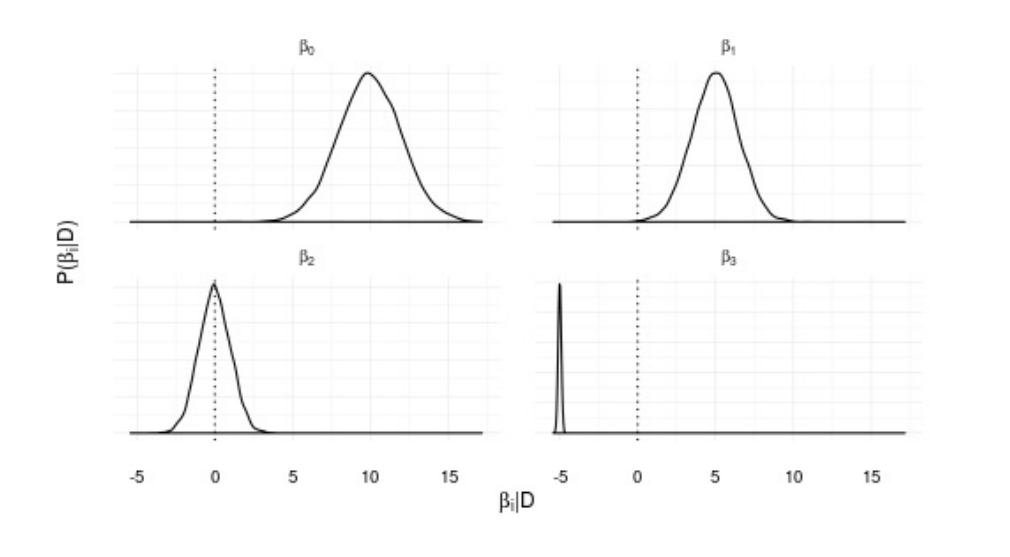Posterior distributions for a Bayesian linear regression. The peak of the distribution represents the most likely value for the parameter, while the spread represents the uncertainty about it.
But unlike frequentists, bayesians can assign a probability to a hypothesis, and compute it directly using Bayes theorem. In this way, we can determine, with a strong basis in theory, the likelihood of a hypothesis given data.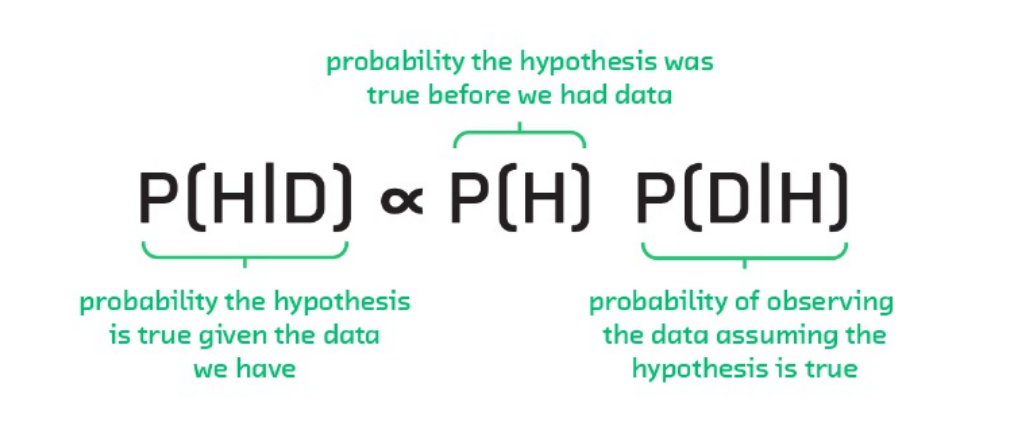Bayes Theorem applied to hypothesis evaluation. (Credit: Fast Forward Labs)

And, unlike supervised learning methods, statistics provide the full distribution of the response variable given the features, allowing us to ask any number of questions related to it. This conditional distribution also encodes the uncertainty about our predictions, allowing us, for example, to compute prediction intervals as opposed to single values for each input combination.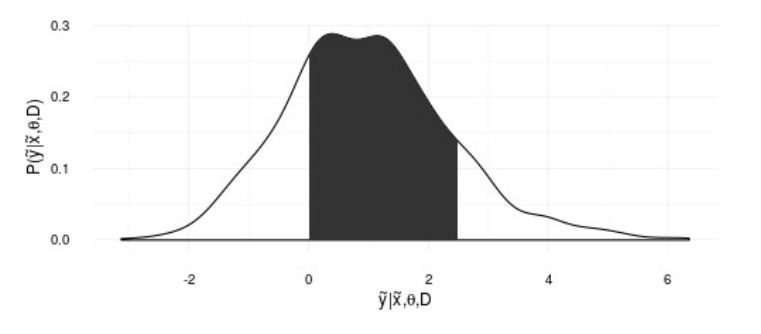The predictive distribution of y given variables x and data D. The shadowed area represents the probability that y is between 0 and 2.5

### Some Limitations

Of course, there is a reason mainstream science uses frequentist methods instead of bayesian ones, and it comes down to practicality; for the past centuries, the applicability of Bayesianism was limited by the hard, sometimes impossible, integrals that must be solved or approximated to make it work. One is needed to compute the “posterior” distribution, that is, the measure of uncertainty after observing data, and another for the predictive distribution, which will tell us what is the likely value of a “new” data point, possibly given some other variables.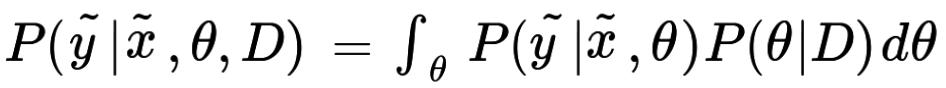The predictive distribution. This integral is often impossible to solve analytically
Fortunately, recent developments in Monte Carlo Markov Chains, have arisen as a way to simulate from these distributions without the need to explicitly compute the integrals. By simulating many observations from the posterior or predictive distributions, we can compute any probability that can be derived from them.

Even more advanced methods, such as Automatic Differentiation Variational Inference (ADVI) further reduce the time and tuning needed to arrive at the posterior distributions.

There are further philosophical questions and practical considerations that have prevented the mainstream use of these methods, although the latter have been somewhat lessened by recent developments in probabilistic programming.

### Probabilistic Programming

Probabilistic programming is the name given to frameworks capable of fully specifying a Bayesian model and make inference with only a couple of lines.

The following snippets are taken from an example of a mean change detection model, taken from the excellent book by Cameron Davidson-Pilon, Bayesian Methods for Hackers, where you can find it in full.

Here is the model specification in PyMC3.

A mean change model using PyMC3
Making inference (i.e. solving those ugly integrals) can also be done in just a couple of lines:

Inference using PyMC3, using the Metropolis MCMC algorithm
While PyMC3 is a great framework, there are many others if Python is not your cup of tea, such as Anglican for Clojure or the standalone Stan.

### Love Uncertainty

In conclusion, Bayesian Statistics provide a framework for data analysis which can overcome many limitations prevalent in different techniques such as Supervised Learning and Frequentist Statistics.

In particular, they provide a way to deal with the problem of overcertainty, allowing us to ask questions about probability, and enabling the analyst to have a healthier relationship with uncertainty, by measuring and presenting it instead of blindly reducing it.

Original. Reposted with permission.

Bio: Adolfo Martínez is a data scientist at datank.ai and a self-confessed professional procrastinator.

Related:

Top Stories Past 30 Days
Most Popular
Most SharedGet KDnuggets, a leading newsletter on AI, Data Science, and Machine Learning